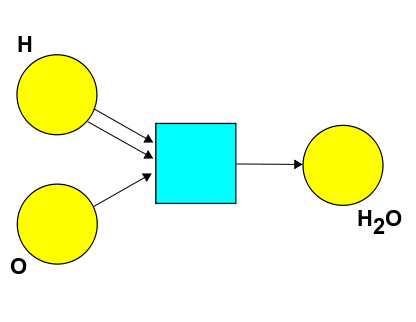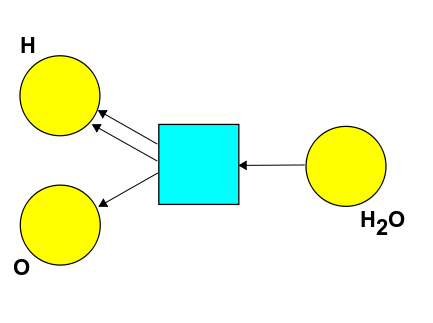Last time we took our beloved preorders and combined them with another kind of mathematical gadget - monoids - to get "monoidal preorders". Preorders say when can get something from something else. Monoids let us combine things. Monoidal preorders let us do both!

This is a common trick in math: taking two mathematical structures and blending or "hybridizing" them to get a new one. It's good to think carefully about it:

1. A **preorder** is a set \$$X\$$ with a relation \$$\le\$$ that's reflexive and transitive.

2. A **monoid** is a set \$$X\$$ where we can combine elements using an associative operation \$$\otimes\$$, together with an element \$$I\$$ that has no effect when we combine it with anything else: \$$I \otimes x = x = x \otimes I\$$.

But to hybridize these, we don't just rudely slap them together. We want our \$$\le\$$ relation to get along with our "combining" operation \$$\otimes\$$! So, we define a **monoidal preorder** to be a thing \$$(X, \le, \otimes, I) \$$ such that \$$(X,\le \$$ is a preorder, \$$(X, \otimes, I) \$$ is a monoid, and also

$x \le x' \textrm{ and } y \le y' \textrm{ imply } x \otimes y \le x' \otimes y' .$

This last condition is the magic [pixie dust](https://en.oxforddictionaries.com/definition/us/pixie_dust) that makes monoidal preorders really interesting.

On the one hand this condition makes perfect sense: if you can get \$$x\$$ from \$$x'\$$ and \$$y\$$ from \$$y'\$$, you should be able to get \$$x\$$ combined with \$$y\$$ from \$$x'\$$ combined with \$$y'\$$.

But on the other hand, you might wonder if it's really "magic". Did finding this condition require a burst of inspiration? Or could there be some systematic way to have found it? Indeed there is!

**Puzzle 66.** What's another way to state this last condition using ideas we've already discussed? Hint: it's a property of the function \$$\otimes: X \times X \to X\$$.

A good category theorist knows lots of systematical ways to find good definitions, which convert "magic" to "method".

A lot of the monoidal preorders will be talking about are "symmetric". This means that given \$$x\$$ combined with \$$y\$$, you can always get \$$y\$$ combined with \$$x\$$.

**Definition.** A monoidal preorder \$$(X, \le, \otimes, I) \$$ is **symmetric** if

$y \otimes x \le x \otimes y$

for all \$$x,y\in X\$$.

Since \$$x\$$ and \$$y\$$ are _anything_, we can switch their roles and conclude that

$y \otimes x \le x \otimes y \textrm{ and } x \otimes y \le y \otimes x$

for all \$$x,y\$$ in a symmetric monoidal preorder. You might guess that this implies \$$x \otimes y = y \otimes x\$$, but no! This will follow if our preorder is a poset, since a poset is a preorder where

$a \le b \textrm{ and } b \le a \textrm{ implies } a = b .$

But it's not true in general:

**Puzzle 67.** A monoidal preorder is **commutative** if \$$x \otimes y = y \otimes x\$$ for all \$$x,y\$$. Find a symmetric monoidal preorder that is not commutative.

There are lots of great examples of symmetric monoidal preorders. For starters, many of your favorite number systems:

1. The set \$$\mathbb{R}\$$ of real numbers with the usual \$$\le\$$, the binary operation \$$+: \mathbb{R} \times \mathbb{R} \to \mathbb{R} \$$ and the element \$$0 \in \mathbb{R}\$$ is a symmetric monoidal preorder.

2. Same for the set \$$\mathbb{Q}\$$ of rational numbers.

3. Same for the set \$$\mathbb{Z}\$$ of integers.

4. Same for the set \$$\mathbb{N}\$$ of natural numbers.

In fact, all these are commutative monoidal posets. But we've also seen some other examples in our discussion of chemistry and Petri nets in [Lecture 19](https://forum.azimuthproject.org/discussion/2079/lecture-19-chapter-2-chemistry-and-scheduling/p1). For example, take this Petri net:This describes the formation of water from hydrogen and oxygen atoms (in an idealized and unrealistic way - it's not really that simple). From this you can create a symmetric monoidal preorder where the elements are expressions like this:

$a \text{H} + b \text{O} + c \text{H}_2\text{O}$

where \$$a,b,c\in \mathbb{N}\$$. Mathematical chemists call these **complexes**. There's an operation for combining complexes, called \$$+\$$, and it's defined this way:

$(a \text{H} + b \text{O} + c \text{H}_2\text{O} ) + (a' \text{H} + b' \text{O} + c' \text{H}_2\text{O} ) = (a+a') \text{H} + (b+b') \text{O} + (c+c') \text{H}_2\text{O}$

This \$$+\$$ operation is associative, and the element

$0 = 0 \text{H} + 0 \text{O} + 0 \text{H}_2\text{O}$

has the property that \$$0 + x = x = x + 0 \$$ for any complex. So, the set of complexes becomes a monoid. The only question is how we define \$$\le\$$.

**Puzzle 68.** How should we define \$$\le\$$ on the set of complexes to get a symmetric monoidal preorder, if the only nontrivial chemical reaction we want to describe is the one shown below?**Puzzle 69.** Is this symmetric monoidal preorder a poset?

**Puzzle 70.** Suppose we _also_ consider the reverse reaction:How should we change our definition of \$$\le\$$ to get a new symmetric monoidal preorder? Is this a poset?

**[To read other lectures go here.](http://www.azimuthproject.org/azimuth/show/Applied+Category+Theory#Chapter_2)**